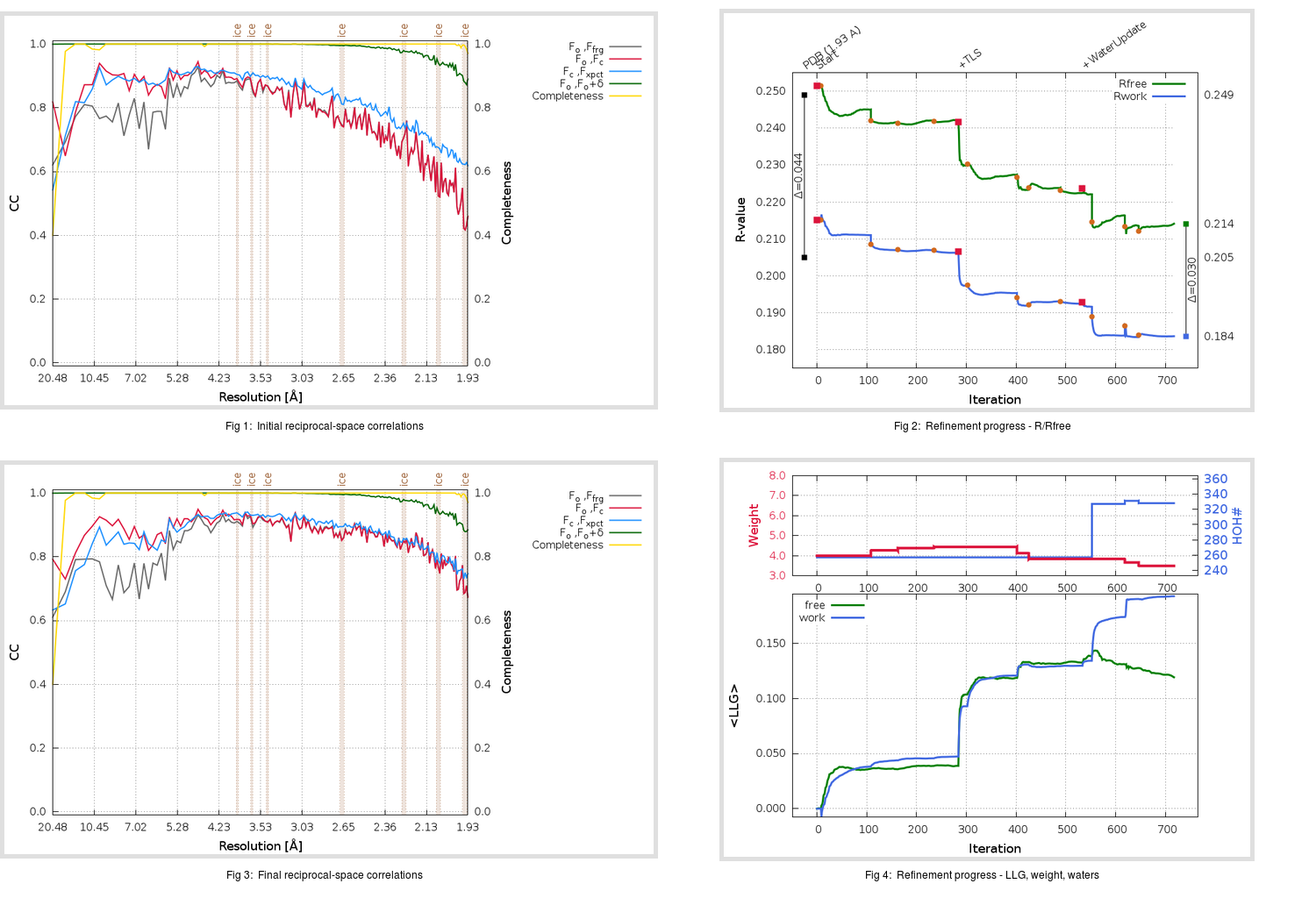Content:

```    Diffraction limits & principal axes of ellipsoid fitted to diffraction cut-off surface:
1.902         0.8775   0.0000   0.4796       0.991 a* + 0.133 c*
1.900         0.0000   1.0000   0.0000       b*
1.858        -0.4796   0.0000   0.8775      -0.711 a* + 0.703 c*
```

## Deposited

` `
 Date deposited Date data collection Resolution R, Rfree 20060508 20050203 1.93 0.2050 0.2490

Molprobity (CCP4 7.0 version) summary:

```Ramachandran outliers =   0.00 %
favored =  97.37 %
Rotamer outliers      =   1.52 %
C-beta deviations     =     0
Clashscore            =   7.74
RMS(bonds)            =   0.0060
RMS(angles)           =   1.43
MolProbity score      =   1.68
Resolution            =   1.93
R-work                =   0.2050
R-free                =   0.2490
```

```Number of waters      =   257

<B> (all atoms) =   39.27 ( sd =   10.89 ) for       2671 non-hydrogen atoms
<B>   (protein) =   38.52 ( sd =   10.71 ) for       2371 non-hydrogen atoms
<B>     (water) =   47.10 ( sd =   10.20 ) for        257 non-hydrogen atoms
<B>    (others) =   33.98 ( sd =    2.82 ) for         43 non-hydrogen atoms

B min/max       (all non-hydrogen atoms) =   18.78 /   78.40
B min/max   (protein non-hydrogen atoms) =   18.78 /   78.40
B min/max     (water non-hydrogen atoms) =   23.44 /   71.87
B min/max     (other non-hydrogen atoms) =   27.73 /   39.54
```

## BUSTER (re-)refinement

` `

Molprobity (CCP4 7.0 version) summary:

```Ramachandran outliers =   0.00 %
favored =  98.36 %
Rotamer outliers      =   3.80 %
C-beta deviations     =     1
Clashscore            =   2.51
RMS(bonds)            =   0.0113
RMS(angles)           =   1.59
MolProbity score      =   1.48
Resolution            =   1.93
R-work                =   0.1837
R-free                =   0.2142
```

```Number of waters      =   328

<B> (all atoms) =   41.88 ( sd =   11.41 ) for       2742 non-hydrogen atoms
<B>   (protein) =   40.33 ( sd =   10.16 ) for       2371 non-hydrogen atoms
<B>     (water) =   54.09 ( sd =   13.02 ) for        328 non-hydrogen atoms
<B>    (others) =   34.15 ( sd =    2.86 ) for         43 non-hydrogen atoms

B min/max       (all non-hydrogen atoms) =   24.26 /  109.39
B min/max   (protein non-hydrogen atoms) =   24.26 /   79.49
B min/max     (water non-hydrogen atoms) =   26.24 /  109.39
B min/max     (other non-hydrogen atoms) =   30.06 /   38.94
```

Refinement progression:Results:

` `
 File Remark 2GX4_aB_refine.01_03_refine.pdb.gz exact refinement commands are in header 2GX4_aB_refine.01_03_refine.mtz.gz including original deposited data and several re-refinement map coefficients 2GX4_aB_refine.01_03_BUSTER_model.cif.gz including any non-standard compound restraints 2GX4_aB_refine.01_03_BUSTER_refln.cif.gz